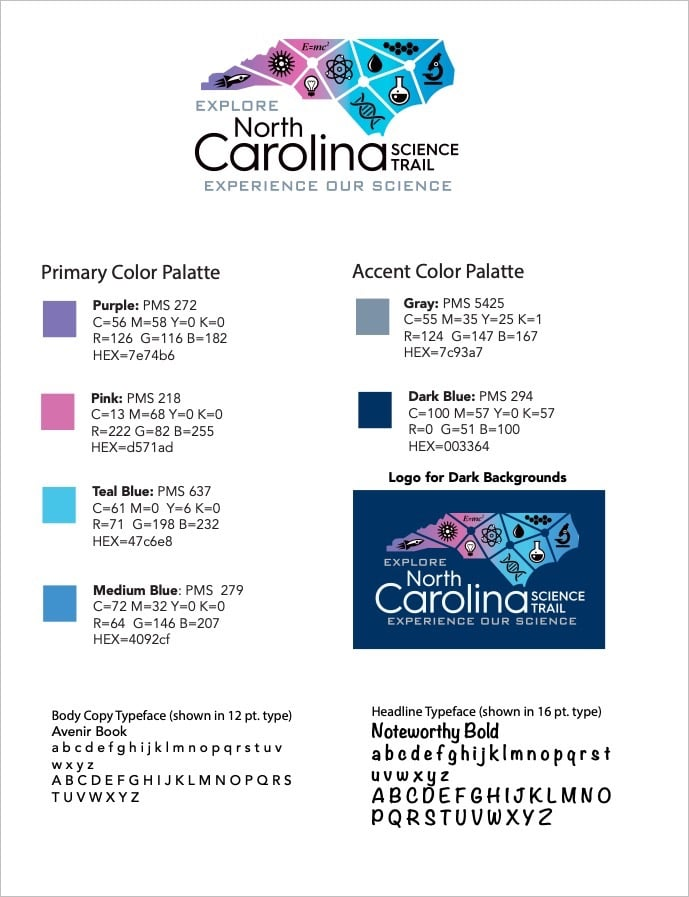Light Background

Dark Background

## Color Guidelines

PMS 272
C=56 M=58 Y=0 K=0
R=126 G=116 B=182 HEX=7e74b6

PMS 218
C=13 M=68 Y=0 K=0
R=222 G=82 B=255

PMS 637
C=61 M=0 Y=6 K=0
R=71 G=198 B=232
HEX=47c6e8

PMS 279
C=72 M=32 Y=0 K=0
R=64 G=146 B=207
HEX=4092cfPMS 5425
C=55 M=35 Y=25 K=1
R=124 G=147 B=167
HEX=7c93a7

PMS 294
C=100 M=57 Y=0 K=57
R=0 G=51 B=100
HEX=003364